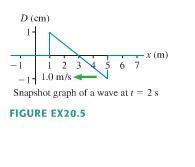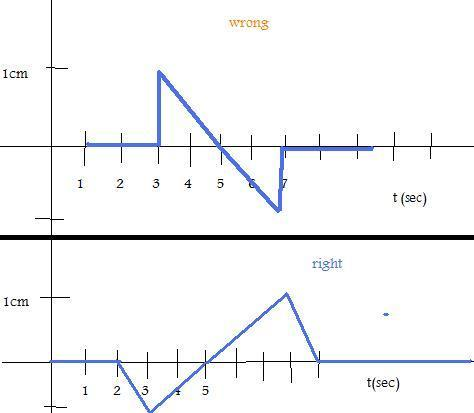# Snapshot & History Graphs

bcjochim07

## Homework Statementfigure is asnapshot.jpgcorrect & incorrect graphs is aasnapshot.jpg

Draw the history graph D(x=0m, t) at x= 0m for the wave shown in the figure.

## The Attempt at a Solution

I'm not quite understanding the concept of snapshot and history graphs. I think for this one the problem is that is is moving left. The graph that I drew is the wrong one. Here was my reasoning: In the figure, the graph is moving left, so shouldn't the leftmost point on the wave hit the point first? If at t=2 s this leftmost point is 1m away from x=0, then it takes 1 sec for it to arrive, so at t=3. I don't think I am visualizing this at all correctly. Could someone please explain to me why the graph on the bottom is correct? Thanks!

bcjochim07
Any thoughts would be greatly appreciated.

bcjochim07
I am confused and this is really bothering me.

Staff Emeritus
Homework Helper

## The Attempt at a Solution

I'm not quite understanding the concept of snapshot and history graphs. I think for this one the problem is that is is moving left. The graph that I drew is the wrong one. Here was my reasoning: In the figure, the graph is moving left, so shouldn't the leftmost point on the wave hit the point first?
Yes.

If at t=2 s this leftmost point is 1m away from x=0, then it takes 1 sec for it to arrive, so at t=3.
Yes, nothing happens (at x=0 m) until t = 3 sec.

I don't think I am visualizing this at all correctly. Could someone please explain to me why the graph on the bottom is correct? Thanks!

It's not correct. At x = 0, D is 0 until t=3 sec. The graph labeled "wrong" looks like the right one to me.

bcjochim07
Yeah, see I'm having problems because there are a couple problems like this in my textbook where my drawings are completely off from what the back of the book has, so I was worried that I was not understanding this at all.

Staff Emeritus
Homework Helper
Wow. What's the name of this book, and the authors? Looks like you understand it better than they do!

freshfruit007
My answer is the same as the original poster's..please, what are we doing wrong?!??!

Staff Emeritus
Homework Helper
Welcome to Physics ForumsYou and the OP did it right, the book has it wrong.

What is the name of this book and its author?

Klorey
The book's called Physics for Scientists and Engineers: A Strategic Approach (2nd ed.), Vol 3, and the author is Randall D. Knight. I also got the same answer as the OP. So the book is wrong and the OP is right?

Staff Emeritus
Homework Helper
Welcome to PFThe book's called Physics for Scientists and Engineers: A Strategic Approach (2nd ed.), Vol 3, and the author is Randall D. Knight. I also got the same answer as the OP. So the book is wrong and the OP is right?
You are correct.

And thank you for answering my questionKlorey
No problemThanks for the help (and the fast reply)!

Epsillon
I have the 6th edition of this book and the answer in the answer key is still wrong...